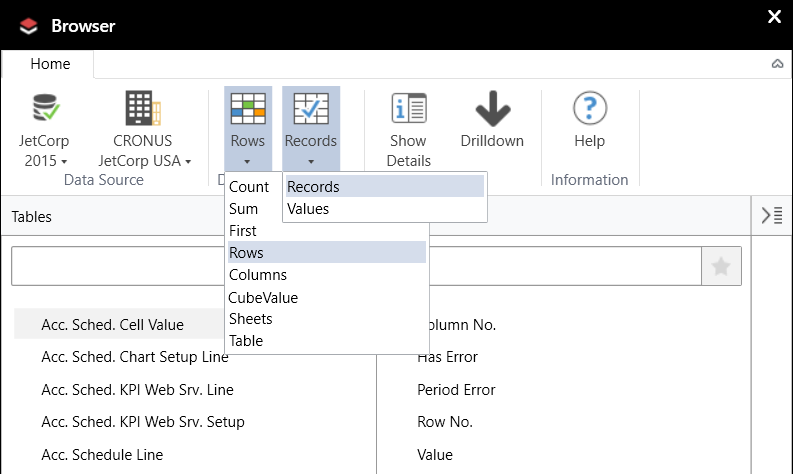Community
Training
Submit a Request
Become a Jet Insider
Give Feedback

# Jet Browser Drop-down Functions

The Jet Browser includes various dropdown settings which determine how the Browser functions:Lists here are the results you can expect, depending upon which settings you choose and whether you drag-and-drop a single table, a single field, or multiple fields:

 Keyword Drop Table Drop Single Field Drop Multiple Fields Count Creates an NL(Count) function that counts the number of records in the table Creates an NL(CountUnique) function that counts the unique values found in the dragged field. N/A Sum N/A Creates an NL(Sum) function that sums the values in the dragged field N/A First Creates an NL(First) formula that displays the first record key in the table. After doing this, you can drag fields onto the same row or column to create NF formulas that retrieve the dragged field from the previously created record. Creates an NL(First) formula that displays the first value in the dragged field. N/A Rows-Records Creates an NL(Rows) formula that lists the record keys in the table. After this, you can drag single fields into the same row to create NF formulas that retrieve the dragged field from the previously listed records. Creates an NL(Rows) formula that lists records from the specified table and an NF formula to retrieve the field value for the dragged field. Creates an NL(Rows) formula that lists the records in the selected table along with NF formulas that retrieve the field values for each of the dragged fields. Rows-Values Same as Records. Creates an NL(Rows) formula that lists the unique values in the dragged field. N/A Columns-Records Same as Rows but creates an NL(Columns) formula. Same as Rows but creates an NL(Columns) formula. Same as Rows but creates an NL(Columns) formula. Columns-Values Same as Rows but creates an NL(Columns) formula. Same as Rows but creates an NL(Columns) formula. N/A CubeValue Creates an NL(CubeValue) formula that returns a single measure value from a Cube data source. Creates an NL(CubeValue) formula that displays the measure value from the dragged measure. N/A Sheets N/A Creates an NL(Sheets) formula that lists unique values of the dragged field. N/A Table Creates an NL(Table) formula that inserts an Excel table which contains a row for every record in the table. All fields will be included in the resulting Excel table. Creates an NL(Table) formula that inserts an Excel table which contains a row for each unique value of the dragged field. Creates an NL(Table) formula that inserts an Excel table which contains a row for each unique combination of the dragged fields.